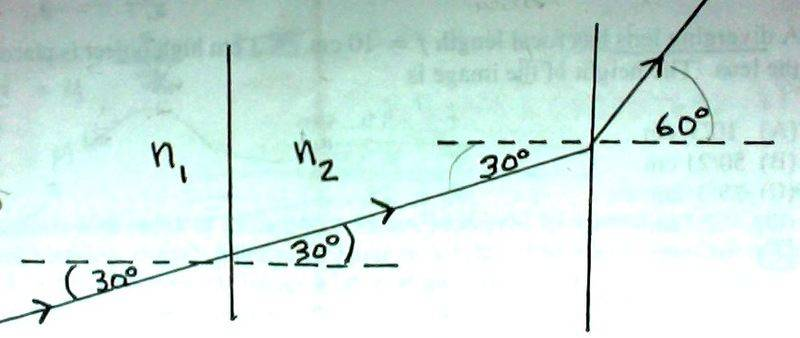# Stressed about Physics Exam! Help with refractive index concept!

7. The figure shows the path of a portion of a ray of light as it passes through three different materials. What can be concluded concerning the refractive indices of these three materials.

(A) n1 < n2 < n3
(B) n1 > n2 > n3
(C) n3 < n1 < n2
(D) n2 = n1 > n3
(E) n1 = n2 < n3According the the diagram above, n1 DOES in fact = n2. The ray of light just goes through each medium without bending or refracting. HOWEVER, once it hits the 3rd material, it bends!
And the angles for n1 and n2 (which are the same) are MUCH smaller than the angle for n3.
I chose E but that's the wrong answer. the Answer is D... why? :(

Does index of refraction refer to the angle of which the light is passing through each material??
I have a physics exam on wednesday and I'm stressed out because I also have a microbiology exam on that same day.
So i have to understand all the answers to the questions I missed on the previous tests to study for this exam

Let's look at this with Snell's law: $n_1sin(θ_1) = n_2sin(θ_2)$. Remember that the θs are measured with respect to the normal from the surface. With that in mind, how can you compare the $n$ values for each of these?

Let's look at this with Snell's law: $n_1sin(θ_1) = n_2sin(θ_2)$. Remember that the θs are measured with respect to the normal from the surface. With that in mind, how can you compare the $n$ values for each of these?

Light (coming out of material n2) bends AWAY from the normal A LOT when it gets to medium/material n3.
This means that there is not as much density in medium n3 to slow light down as there is in materials n1 and n2 (which are, according to the pic, as dense as each other, but more dense than material 3).

So the concept is...the bigger the angle = the bigger the index of refraction (ie. light bends easily)?

Or I should say...

The index of refraction measures the bending of a ray of light when passing from 1 medium to another.

The thicker the medium - the greater the "n", the slower the light passes, the smaller the angle (more towards the normal)
The thinner the medium - the smaller the "n", the faster the light passes, the larger the angle (more away from the normal)

Right?

You still there? :(

Look at how the quantities are related in Snell's Law: (ie. $n_1sin(θ_1) = n_2sin(θ_2)$)

If $n_2$ gets larger, then $θ_2$ gets smaller.
If $n_2$ gets smaller, then $θ_2$ gets larger.

So from the angle of the refracted ray, you are able to reason out the relative indices of refraction.

Let's step back from the physicality for a minute (we'll come back to it), and just follow Snell's law, which xatu and I have stated earlier. Applying this to the first barrier, we get:
$$n_1sin(30°) = n_2sin(30°)\\n_1 = n_2$$
And we come to the first conclusion, which you came up with conceptually. Does this process make sense to you? If so, go ahead and apply it to the second barrier. If not, we'll need to review Snell's law; it should have been mentioned in your class, and it'd be a good thing to know before the test.

Let's step back from the physicality for a minute (we'll come back to it), and just follow Snell's law, which xatu and I have stated earlier. Applying this to the first barrier, we get:
$$n_1sin(30°) = n_2sin(30°)\\n_1 = n_2$$
And we come to the first conclusion, which you came up with conceptually. Does this process make sense to you? If so, go ahead and apply it to the second barrier. If not, we'll need to review Snell's law; it should have been mentioned in your class, and it'd be a good thing to know before the test.

Ohh I get it.

So

$$n_2sin(30°) = n_3sin(60°)\\n_1 = n_2$$

For n2, θ2 is small, so n2 must be larger.
For n3, θ3 is large, so n3 must be smaller.

Thanks!!

I have just a few more questions regarding index of refractions and reflections...

8. A fly is 10 cm above the surface of a pond. How high above the water does the fly appear to a fish directly below (nair = 1, nwater = 1.333)?

(A) 10 cm
(B) 7.5 cm
(C) 13.3 cm
(D) 12.5 cm
(E) fish cannot see the fly because the light is totally reflected

The answer is supposed to be C but how did they figure this out?
They gave me height (h = 10 cm), but I have no angles of refraction, no just the index of refractions. But I have no formula to solve for HEIGHT because I can't use Snell's Law Equation. This makes no sense :(

9. What angle of incidence in degrees is sunlight 100% polarized when reflecting from the surface of the pond in the previous question?

(A) 0º
(B) 53º
(C) 37º
(D) 45º
(E) there is no angle

The answer is B, but how?

Anybody still willing to help me out? :(